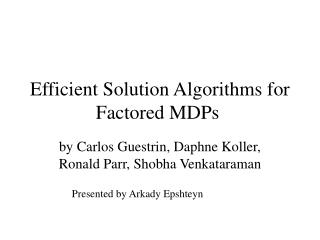DownloadDownload PresentationEfficient Solution Algorithms for Factored MDPs

# Efficient Solution Algorithms for Factored MDPs

Download Presentation## Efficient Solution Algorithms for Factored MDPs

- - - - - - - - - - - - - - - - - - - - - - - - - - - E N D - - - - - - - - - - - - - - - - - - - - - - - - - - -
##### Presentation Transcript

1. Efficient Solution Algorithms for Factored MDPs by Carlos Guestrin, Daphne Koller, Ronald Parr, Shobha Venkataraman Presented by Arkady Epshteyn

2. Problem with MDPs • Exponential number of states • Example: Sysadmin Problem • 4 computers: M1, M2 , M3 , M4 • Each machine is working or has failed. • State space: 24 • 8 actions: whether to reboot each machine or not • Reward: depends on the number of working machines

3. Factored Representation • Transition model: DBN • Reward model:

4. Approximate Value Function • Linear value function: • Basis functions: hi(Xi=true)=1 hi(Xi=false)=0 h0=1

5. Markov Decision Processes For fixed policy : The optimal value function V*:

6. Solving MDPMethod 1: Policy Iteration • Value determination • Policy Improvement • Polynomial in the number of states N • Exponential in the number of variables K

7. Solving MDPMethod 2: Linear Programming • Intuition: compare with the fixed point of V(x): • Polynomial in the number of states N • Exponential in the number of variables

8. Value Function Approximation

9. Objective function • Objective function polynomial in the number of basis functions

10. Each Constraint: Backprojection

11. Representing Exponentially Many Constraints

12. Restricted Domain 1 2 3 • Backprojection - depends on few variables • Basis function • Reward function

13. Variable Elimination - similar to Bayesian Networks

14. Maximization as Linear Constraints • Exponential in the size of each function’s • domain, not the number of states

15. Factored LP: Scaling

16. Rule-based Representation

17. Approximate Value Function x1 h1: x3 0 5 0.6 Notice: compact representation (2/4 variables, 3/16 rules)

18. Summing Over Rules x2 h1(x) h2(x) x1 x1 x2 x1 = + u1+u4 x3 u5+u1 x3 x1 x3 u4 u1 u3+u4 u2+u4 u5 u6 u2 u3 u2+u6 u3+u6

19. Multiplying over Rules • Analogous construction

20. Rule-based Maximization x1 x1 Eliminate x2 x3 x2 u1 u1 u2 x3 max(u2,u3) max(u2,u4) u3 u4

21. Rule-based Linear Program • Backprojection, objective function – handled in a similar way • All the operations (summation, multiplication, maximization) – keep rule representation intact • is a linear function

22. Conclusions • Compact representation can be exploited to solve MDPs with exponentially many states efficiently. • Still NP-complete in the worst case. • Factored solution may increase the size of LP when the number of states is small (but it scales better). • Success depends on the choice of the basis functions for value approximation and the factored decomposition of rewards and transition probabilities.